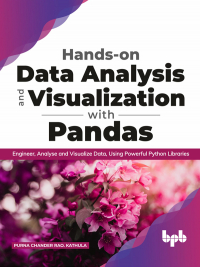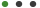# Hands-on Data Analysis and Visualization with Pandas

Formats - EPUB, PDF

Pages - 316

ISBN - 9789389845648#### Description

Learn how to use JupyterLab, Numpy, pandas, Scipy, Matplotlib, and Seaborn for Data science

## Key Features

â— Get familiar with different inbuilt Data structures, Functional programming, and Datetime objects.
â— Handling heavy Datasets to optimize the data types for memory management, reading files in chunks, dask, and modin pandas.
â— Time-series analysis to find trends, seasonality, and cyclic components.
Seaborn to build aesthetic plots with high-level interfaces and customized themes.
â— Exploratory data analysis with real-time datasets to maximize the insights about data.

## Description

The book will start with quick introductions to Python and its ecosystem libraries for data science such as JupyterLab, Numpy, Pandas, SciPy, Matplotlib, and Seaborn.
This book will help in learning python data structures and essential concepts such as Functions, Lambdas, List comprehensions, Datetime objects, etc. required for data engineering. It also covers an in-depth understanding of Python data science packages where JupyterLab used as an IDE for writing, documenting, and executing the python code, Numpy used for computation of numerical operations, Pandas for cleaning and reorganizing the data, handling large datasets and merging the dataframes to get meaningful insights. You will go through the statistics to understand the relation between the variables using SciPy and building visualization charts using Matplotllib and Seaborn libraries.

## What will you learn

â— Learn about Python data containers, their methods, and attributes.
â— Learn Numpy arrays for the computation of numerical data.
â— Learn Pandas data structures, DataFrames, and Series.
â— Learn statistics measures of central tendency, central limit theorem, confidence intervals, and hypothesis testing.
â— A brief understanding of visualization, control, and draw different inbuilt charts to extract important variables, detect outliers, and anomalies using Matplotlib and Seaborn.

## Who this book is for

This book is for anyone who wants to use Python for Data Analysis and Visualization. This book is for novices as well as experienced readers with working knowledge of the pandas library. Basic knowledge of Python is a must.

1. Introduction to Data Analysis
2. Jupyter lab
3. Python overview
4. Introduction to Numpy
5. Introduction to Pandas
6. Data Analysis
7. Time-Series Analysis
8. Introduction to Statistics
9. Matplotlib
10. Seaborn
11. Exploratory Data Analysis

No Datials Available